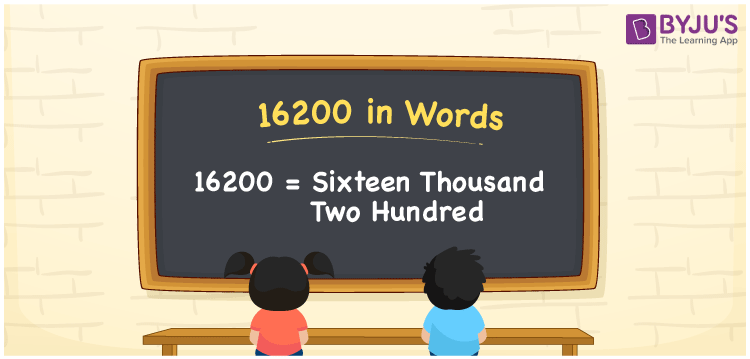# 16200 in Words

16200 in words is written as “Sixteen Thousand Two Hundred”. For example, Rs.16200 is written as “Rupees Sixteen Thousand Two Hundred only” in a cheque. In Maths, 16200 is a cardinal number that expresses a quantity or a value. 16200 can represent any value, like the population of a town, the number of employees in a company, 16200 in dollars or any other currency. Learn more about Numbers In Words and writing the number names in English at BYJU’S.

 16200 in Words Sixteen Thousand Two Hundred Sixteen Thousand Two Hundred in Numbers 16200

## 16200 in English Words## How to Write 16200 in Words?

The number 16200 in words can be written using a place value chart. Since 16200 is a five-digit number, thus, we can represent the digits using the table given below.

 Ten-thousands Thousands Hundreds Tens Ones 1 6 2 0 0

From the above table,

1 → Ten Thousands

6 → Thousands

2 → Hundreds

0 → Tens

0 → Ones

Hence, when we read the number 16200 from right to left, it is Sixteen Thousand Two Hundred.

### Expanded Form of 16200

We can write the expanded form as:

1 x Ten thousand + 6 x Thousand + 2 × Hundred + 0 × Ten + 0 × One

= 1 x 10000 + 6 x 1000 + 2 × 100 + 0 × 10 + 0 × 1

= 1 x 10000 + 6000 + 200 + 0 + 0

= Sixteen Thousand Two Hundred

16200 is a whole number that is succeeded by 16199 and preceded by 16201. Learn more about the number 16200 below:

• 16200 in Words – Sixteen Thousand Two Hundred
• Is 16200 an odd number? – No
• Is 16200 an even number? – Yes
• Is 16200 a perfect square number? – No
• Is 16200 a perfect cube number? – No
• Is 16200 a prime number? – No
• Is 16200 a composite number? – Yes

## Frequently Asked Questions on 16200 in words

Q1

### What is 16200 in words?

16200 in words is given by Sixteen Thousand Two Hundred.
Q2

### What is the rule to write 16200 in words?

To write the 16200 in words, we should know the place value of all the digits.

1 → Ten Thousands

6 → Thousands

2 → Hundreds

0 → Tens

0 → Ones

Thus, the number is read as Sixteen Thousand Two Hundred .

Q3

### What is the value of 16200 + 100 in words?

16200 + 100 = 16300, i.e., Sixteen thousand three hundred in words.